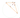## 双心三角形的心迹

Sun, 17th November 2019Edit on Github双心三角形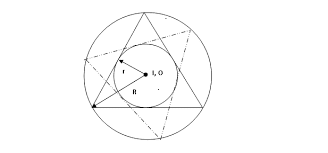mathe在2019年3月玩Geogebra时发现，连接双心三角形顶点和对边于内切圆的切点可以交于一点，而这个点的轨迹通常很小，但是看起来很“圆”。 那么这个轨迹到底是不是圆 呢？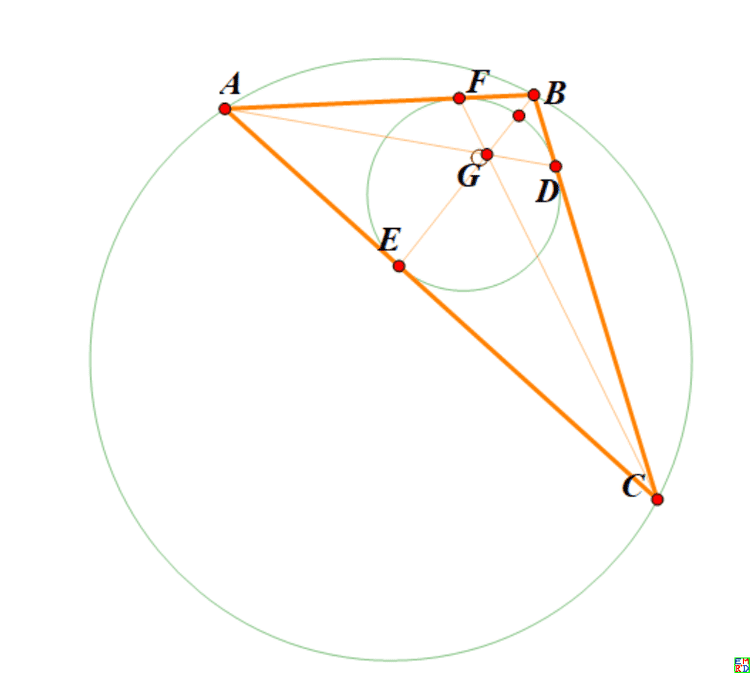# 详细内容

lsr314首先指出，如果轨迹是圆，那么三角形绕一圈时，轨迹会绕三周，而不仅仅一周。zeroieme通过符号运算得出一个及其复杂的表达式， 因式分解得出三个二次表达式的乘积，lsr314给出了对应轨迹图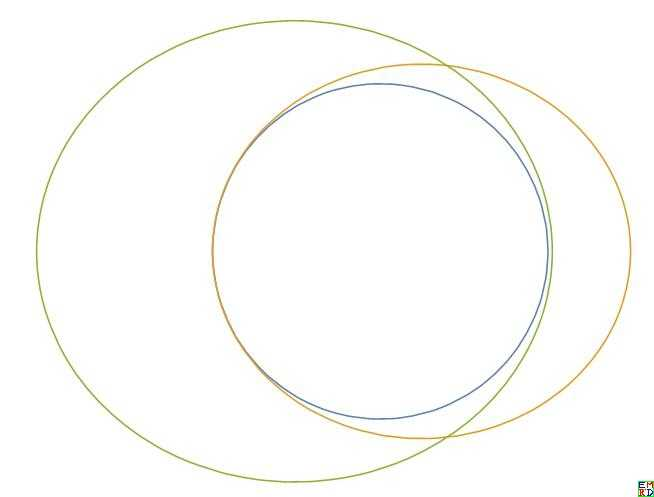wayne在Geogebra下将轨迹局部放大 ，验证结果还是很圆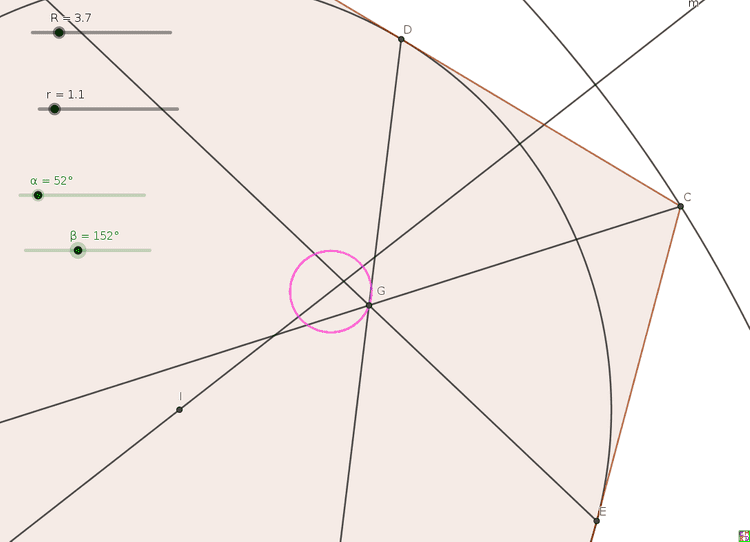lsr314尝试这将双心三角形扩展为双心五边形 ，不过可惜发现顶点和对边切点连线不共点。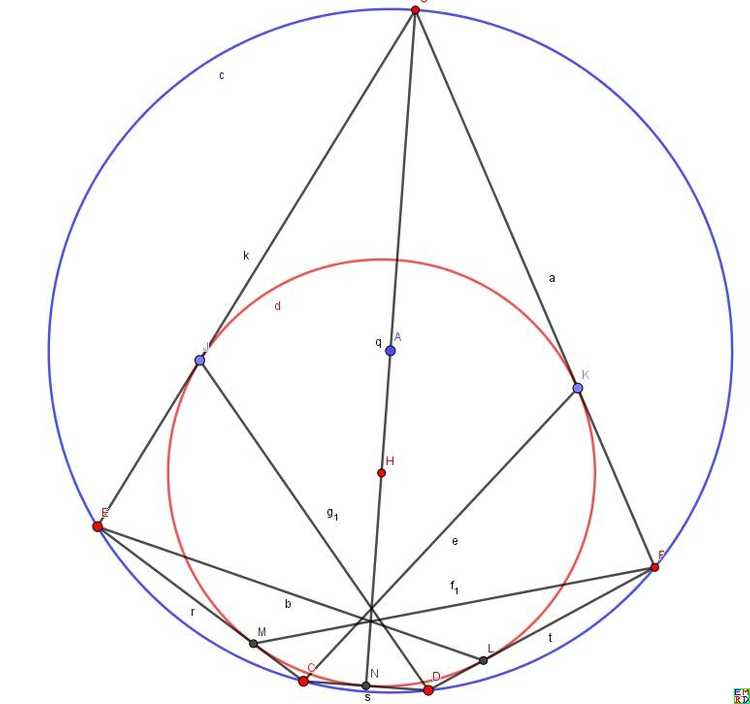wayne认为，我们的不变量有三个: 外接圆，内切圆的半径$R,r$，圆心距$d$依赖于$R,r$, 变量是$A$点的坐标。我们将所有的量尽可能的转化成这三个不变量,以及变量$A$来表达。 运用梅涅劳斯定理，$△ACF$被直线$EGB$切割，于是有：$\frac{AE}{EC}\frac{CG}{GF}\frac{FB}{BA} =1$ ,也就是说$\frac{CG}{GF} = \frac{AB\times CD}{AF\times BF} = \frac{c(s-c)}{(s-a)(s-b)}, s=(a+b+c)/2,$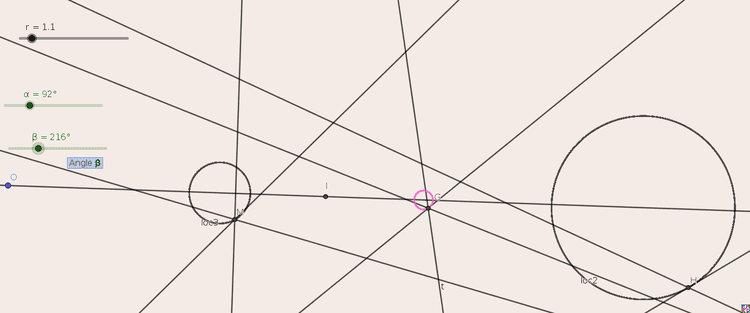lsr314试验了在三角形内分别作三个与内切圆和两边相切的圆，连接对应的切点的三条直线交于一点。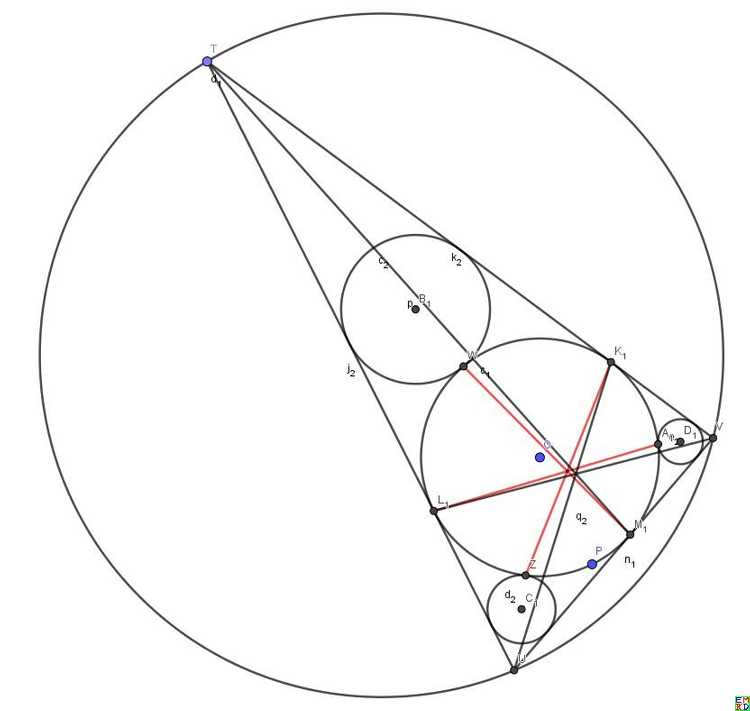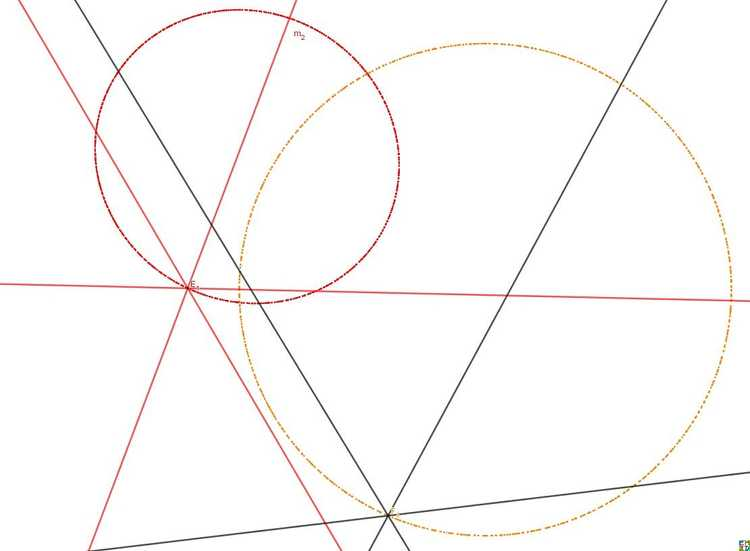lsr314认为三角形欧拉线上的点（相对于外心、重心、垂心的位置不变）的轨迹都是一个圆，这些圆的位似中心就是三角形的外心。 但是反过来不成立，比如旁心的轨迹也是一个圆，但是旁心一般不在欧拉线上。
mathe通过射影变换改变无穷远直线为一条和外圆相交的直线 ，每边中点改为对应的调和共轭点， 结果得到的图几何作图验证一条圆锥曲线，但是不是圆。 开始mathe认为在原图中重心轨迹还是可能是圆（但是也可能是椭圆），但是肯定和原先的两圆不共享一条根轴（也就是不在同一个圆系）。但是后来发现这个图 恰恰说明了重心轨迹的确是圆，因为轨迹经过直线（对应原无穷远直线）和圆的交点，对应于无穷远直线和所有圆相交的两个虚数点(1:i:0), (1:-i:0)。他认为双心三角形中内接圆改为内接椭圆后结论还可能成立。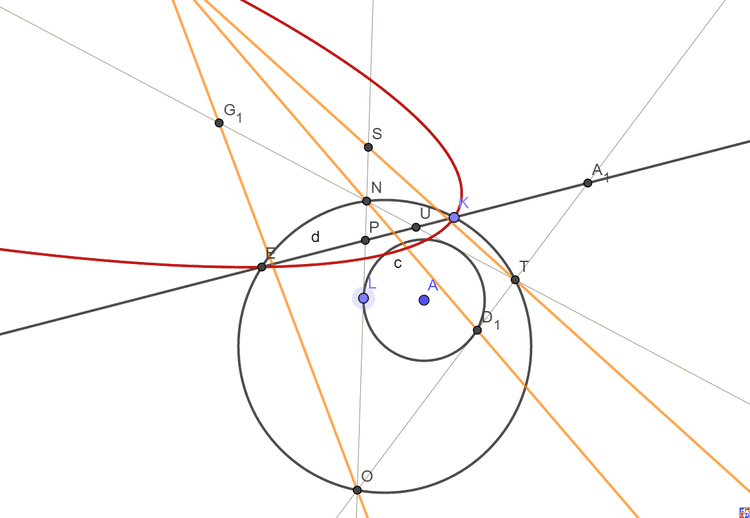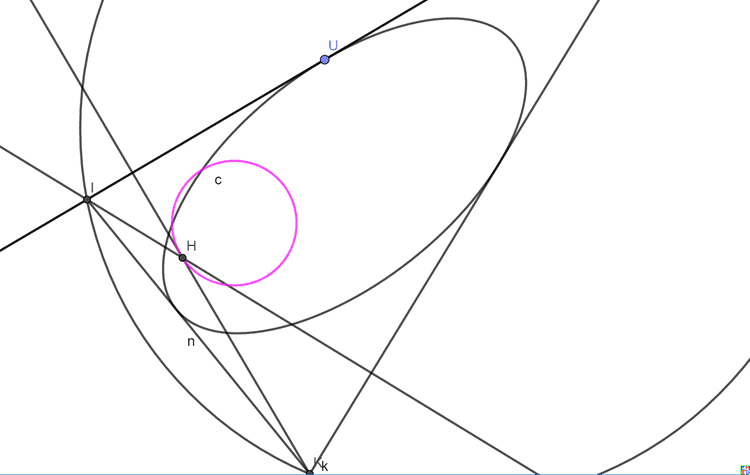mathe在一个图中做出了垂心(H)、重心(G)、旁心(P)、费马尔点(F)和顶点切点连线交点(T)等的轨迹 ，可以看出除了费马尔点其余的都像圆，而费马尔点的轨迹像椭圆mathe建议采用面积坐标表示各心的坐标，并且将边长作为参数，数学星空给出了各心的面积坐标表达式

1.重心$G(1:1:1)$

2.内心$I(a,b,c)$

3.旁心$I_A(-a,b,c),I_B(a,-b,c),I_C(a,b,-c)$

4.外心$O(\sin(2A):\sin(2B):\sin(2C))=a^2(b^2+c^2-a^2):b^2(a^2+c^2-b^2):c^2(a^2+b^2-c^2)$

5.垂心$H(\tan(A):\tan(B):\tan(C)=\frac{1}{b^2+c^2-a^2}:\frac{1}{-b^2+c^2+a^2}:\frac{1}{b^2-c^2+a^2}$

6.九点圆圆心$N(a\cos(B-C):b\cos(C-A):c\cos(A-B))=a^2(b^2+c^2)-(b^2-c^2)^2:b^2(c^2+a^2)-(c^2-a^2)^2:c^2(a^2+b^2)-(a^2-b^2)^2$

7.共轭重心$K(a^2:b^2:c^2)$

8.热尔岗点Gergonne $Ge(\frac{1}{s-a}:\frac{1}{s-b}:\frac{1}{s-c})$

9.奈格尔点(Nagel) $Na(s-a,s-b,s-c)$

10.费尔巴哈点（Feuerbach)$a(1-\cos(B-C)):b(1-\cos(C-A)):c(1-\cos(A-B))$

11.费马点$F(\frac{1}{\cot(A)+\cot(\frac{\pi}{3})}:\frac{1}{\cot(B)+\cot(\frac{\pi}{3})}:\frac{1}{\cot(C)+\cot(\frac{\pi}{3})})$

lsr314还发现双心四边形的重心的轨迹 好像也是圆，但是双心五边形就不行了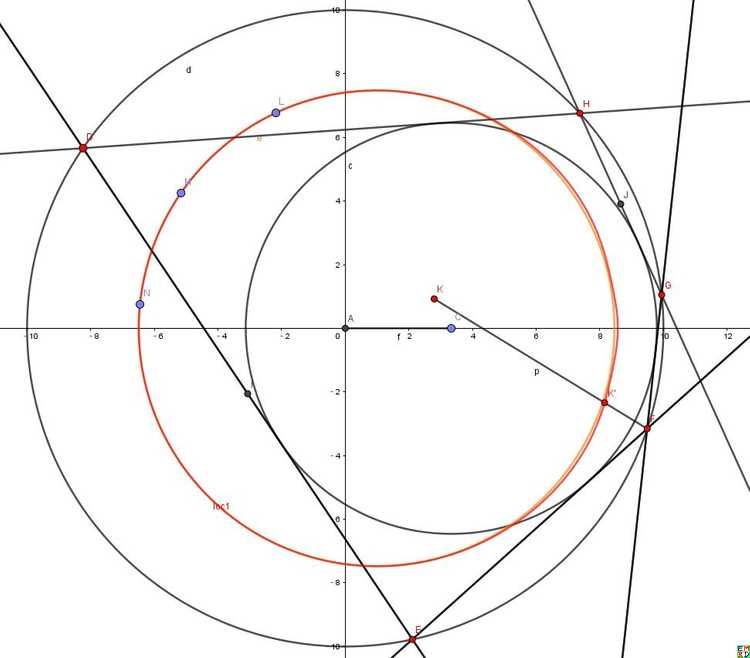mathe提议

1.对于重心G,我们可以设

$x+yI=\frac{A+B+C}{3},x_0+y_0I=\frac{aA+bB+cC}{a+b+c},0=a^2(b^2+c^2-a^2)A+b^2(a^2-b^2+c^2)B+c^2(a^2+b^2-c^2)C$

2.对于垂心H，我们可以得到：

3.对于热尔岗点Ge,我们可以得到：

4.对于奈格尔点Na,我们可以得到：

5.对于九点圆圆心N,我们可以得到：

Github